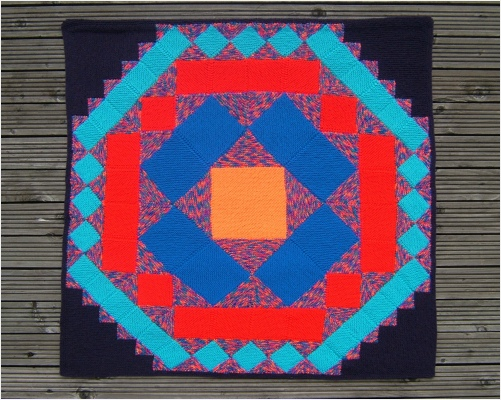﻿ Pythagorean RipplesBuy nowOther places to visit

Order Form

Mental Blocks

The World of Illusion Knittingfor this afghan

on the order formBuy nowPYTHAGOREAN RIPPLES

Every empty edge of each square forms the base of a triangle. There is a square on each empty edge of the new triangles and this pattern ripples outwards, theoretically going on for ever. Based on Pythagoras theorem.

You might call this Pythagoras Gone Mad. It was inspired by the work of a young teacher who gave his pupils a diagram of the centre of this design and asked them to complete it. They soon realised it was impossible to complete as they had to add ever smaller pieces and, if they had the physical means, could keep going for ever.KNITTING INFORMATION

Four main colours are needed plus an extra colour for the background and another if you want to turn it into a square, rather than an afghan with a saw-tooth edge.

There are 65 squares and 108 triangles, each in 4 different sizes.

Any type of yarn can be used.Pythagorean RipplesRELATED DESIGNS

PYTHAGORAS AFGHAN

Pythagoras Tree

PYTHAGORAS CUSHION

The Other Two SidesCONSTRUCTION INFORMATION

The squares are knitted individually and the triangles are knitted on to them by picking up stitches.

The pieces are then joined together.Click to see

larger pictures

The design was inspired by Don’t let anyone tell you Maths isn’t beautiful - an article by Richard Goodman in the March 2000 edition of Mathematics Teaching.

Richard describes how he gave his pupils this task.

1. Draw a square.

2. On each side of the square draw a right angled isosceles triangle such that the hypotenuse of the triangle is the side of the square.

3. On each exposed side of a triangle draw a square.

4. On each exposed side of a square draw a triangle.

5. Go to step 3.

Each stage of the design was used for work relating to the areas of the combined shapes.

Richard goes on to describe the arguments between his pupils over such matters as whether this pattern would ever come to an end, whether a pencil would have enough lead to finish it and how it should be coloured.

He could also have used this as a multiple example of Pythagoras’ Theorem - the large square on one side of a triangle being equal in area to the sum of the two squares on the other two sides. (In knitting terms - as the squares get smaller each size only takes half as long to knit as the one before.)

There is also a great deal of Maths to be found in looking at the relative sizes of the squares and why it is so easy to work out the correct number of stitches needed for each section, to make the pieces fit together.